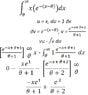# Help with integration

• confused88

## The Attempt at a Solution

u = x, du = 1 dx, dv = e^(-x-theta)) v = e^-x+theta+1 / theta + 1

the rest can be seen in the attachment. Can you please check it out, it would be greatly appreciated.Thanks

#### Attachments

•integration.jpg
27 KB · Views: 322
The attachment is pending approval so I can't see it.

Use the substitution $$u = -x - \theta \Rightarrow x = -(u+\theta) \Rightarrow dx = -du$$. So our integral is now $$\int (u + theta)e^u du$$.

Now use integration by parts.

ohhh wow thanks a lot!

The first thing I would do is write $e^{-x- \theta}= e^{-x}e^{-\theta}$ and factor out the $e^{-\theta}$. Your integral is just $e^{-\theta}\int xe^{-x}dx$ which can be done by integration by parts.